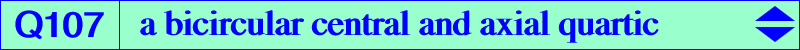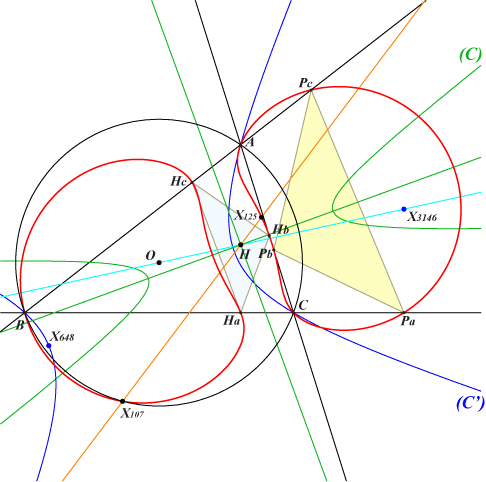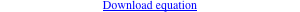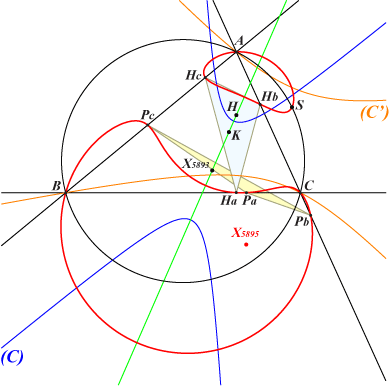too complicated to be written here. Click on the link to download a text file.X(107), X(125) Ha, Hb, Hc : vertices of the orthic triangle Pa, Pb, Pc : vertices of the pedal triangle of X(3146) other points belowQ107 is the locus of P whose orthotransversal passes through X(3146), the image of H under h(O, 3). Q107 in invariant under the following transformations : reflection about the orthocenter H, reflections about the parallels at H to the asymptotes of the Kiepert hyperbola or equivalently to the axes of the Steiner ellipses, orthoassociation i.e. inversion in the polar circle. It follows that Q107 contains the images of the 8 points above under these transformations and, in particular, the reflections of the vertices of the triangles ABC, HaHbHc about H. Q107 is a bicircular quartic, the envelope of circles orthogonal to the polar circle whose center lies on the conic (C) with center H passing through the midpoints of the cevians of X(3146). This conic is called "déférente" in French. Q107 is also the anti-orthocorrespondent of the circum-conic (C') with perspector X(3146). In other words, each point on (C') has two anti-orthocorrespondents on Q107 collinear with H. These two latter points are said to be orthoassociates. For example, the anti-orthocorrespondents of X(648) are X(107), X(125). Construction : A variable line through X(3146) meets the sidelines of ABC at U, V, W. The circles with diameters AU, BV, CW are in a same pencil and meet at two orthoassociate points M1, M2 on Q107. The line passing through their centers (Newton line) is tangent to (C) and the contact is the center M of the circle orthogonal to the polar circle as above. Generalization : If X(3146) is replaced with a point P, the corresponding locus is generally also a bicircular quartic Q(P) but the symmetries above are generally no longer valid (see below). The center of the déférente (C) is ccP, the complement of the complement of P. The construction is the same as that of Q107. Note that two antipodes M, N on (C) correspond to four collinear points on Q(P), two by two orthoassociates on a line through H. When P lies at infinity, the quartic splits into the line at infinity and a circular isogonal pK with pivot H with respect to the orthic triangle as in CL019. Special cases : • with P = X(3), Q(P) splits into the circumcircle and the nine point circle. (C) is the conic with center X(140) passing through the midpoints of the cevians of X(3). Q(P) is obviously symmetric about the Euler line. • with P = X(8), (C) is the Spieker circle with center X(10) and, through extraversion, we obtain the only four points P such that (C) is a circle. • with P = X(5895), Q(P) is symmetric about the line X(4)X(6).Q(X5895) meets the circumcircle again at S, the reflection of X(1301) about the Euler line. S lies on the lines X(4), X(2693) - X(24), X(477) - X(25), X(2697) - X(28), X(2694) - X(74), X(403), etc. The center of the déférente is X(5893), the circumcenter of the half-altitude triangle.